Next: Rotation Operators in Spin Up: Spin Angular Momentum Previous: Properties of Spin Angular

# Wavefunction of Spin One-Half Particle

The state of a spin one-half particle is represented as a vector in ket space. Let us suppose that this space is spanned by the basis kets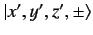. Here,denotes a simultaneous eigenstate of the position operators,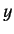,, and the spin operator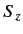, corresponding to the eigenvalues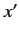,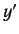,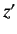, and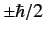, respectively. The basis kets are assumed to satisfy the completeness relation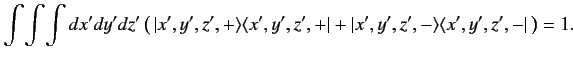(431)

It is helpful to think of the ket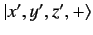as the product of two kets--a position space ket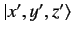, and a spin space ket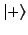. We assume that such a product obeys the commutative and distributive axioms of multiplication: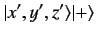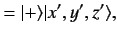(432)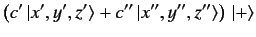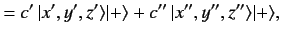(433)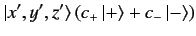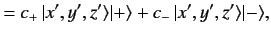(434)

where the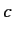's are numbers. We can give meaning to any position space operator (such as) acting on the product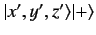by assuming that it operates only on thefactor, and commutes with thefactor. Similarly, we can give a meaning to any spin operator (such as) acting onby assuming that it operates only on, and commutes with. This implies that every position space operator commutes with every spin operator. In this manner, we can give meaning to the equation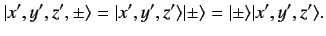(435)

The multiplication in the above equation is of a quite different type to any that we have encountered previously. The ket vectorsand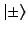lie in two completely separate vector spaces, and their product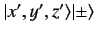lies in a third vector space. In mathematics, the latter space is termed the product space of the former spaces, which are termed factor spaces. The number of dimensions of a product space is equal to the product of the number of dimensions of each of the factor spaces. A general ket of the product space is not of the form (435), but is instead a sum or integral of kets of this form.

A general stateof a spin one-half particle is represented as a ket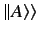in the product of the spin and position spaces. This state can be completely specified by two wavefunctions: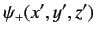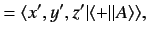(436)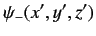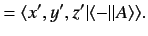(437)

The probability of observing the particle in the regionto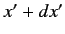,to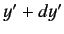, andto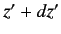, with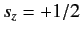is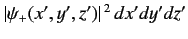. Likewise, the probability of observing the particle in the regionto,to, andto, with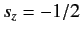is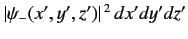. The normalization condition for the wavefunctions is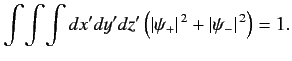(438)Next: Rotation Operators in Spin Up: Spin Angular Momentum Previous: Properties of Spin Angular
Richard Fitzpatrick 2013-04-08